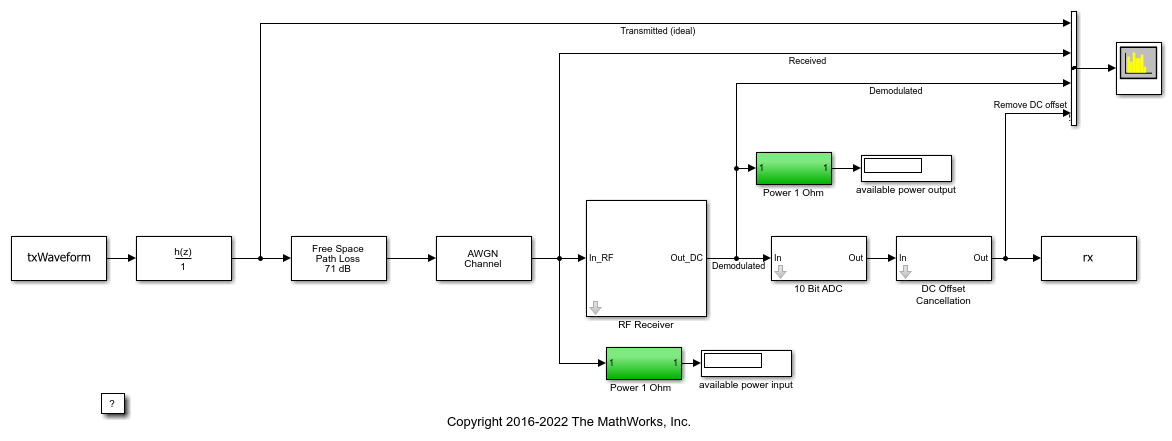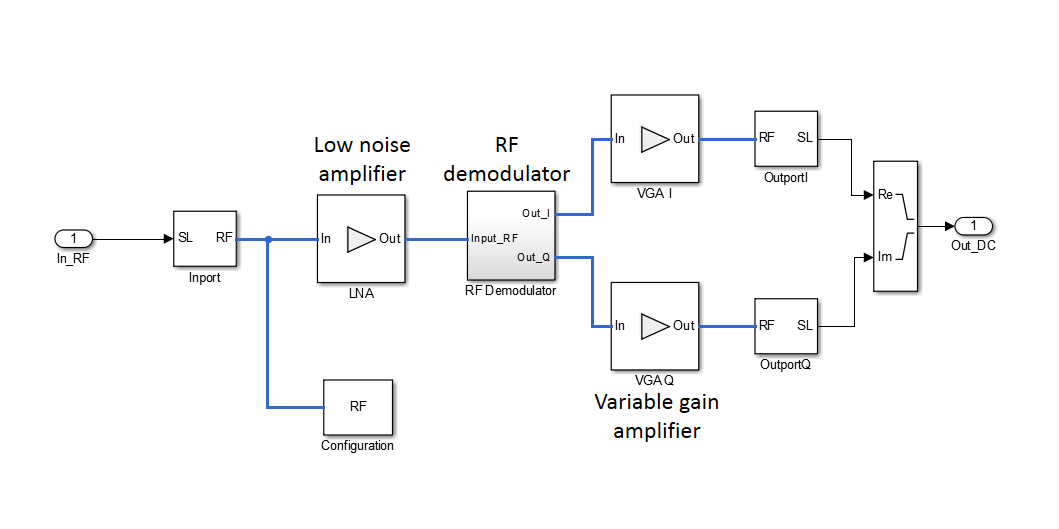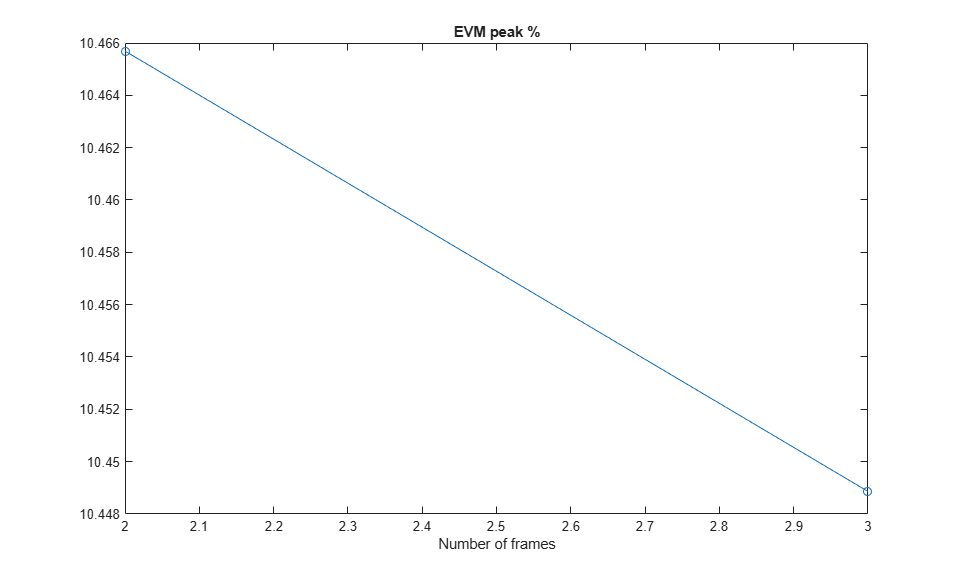# Modeling and Testing an LTE RF Receiver

This example demonstrates how to model and test an LTE RF receiver using LTE Toolbox™ and RF Blockset™.

### Model Description

The figure below shows the main parts of this example. An LTE waveform is generated using the LTE Toolbox. This waveform is filtered and transmitted through a propagation channel before feeding it to the RF receiver model implemented with RF Blockset. This model is based on commercially available parts. EVM figures are then provided for the output of the RF receiver.This example is implemented using MATLAB® and Simulink®, which interact at runtime. The functional partition is shown in the figure belowThe MATLAB script implements the simulation test-bench, and the Simulink model is the device under test (DUT). LTE frames are streamed between the test-bench and the DUT.

### Generate LTE Waveform

In this section we generate the LTE waveform using the LTE Toolbox. We use the reference measurement channel (RMC) R.6 as defined in TS 36.101 [ 1 ]. This RMC specifies a 25 resource elements (REs) bandwidth, equivalent to 5 MHz. A 64 QAM modulation is used. All REs are allocated. Additionally, OCNG noise is enabled in unused REs.

Only one frame is generated. This frame will then be repeated a number of times to perform the EVM measurements.

```% Configuration TS 36.101 25 REs (5 MHz), 64-QAM, full allocation rmc = lteRMCDL('R.6'); rmc.OCNGPDSCHEnable = 'On'; % Create eNodeB transmission with fixed PDSCH data rng(2); % Fixed random seed (arbitrary) data = randi([0 1], sum(rmc.PDSCH.TrBlkSizes),1); % Generate 1 frame, to be repeated to simulate a total of N frames [tx, ~, info] = lteRMCDLTool(rmc, data); % 1 frame % Calculate the sampling period and the length of the frame. SamplePeriod = 1/info.SamplingRate; FrameLength = length(tx); ```

### Initialize Simulation Components

This section initializes some of the simulation components:

• Band limiting filter: design the filter coefficients, which will be used by the Simulink model. The filter has order 32, with passband frequency equal to 2.25 MHz, and stopband frequency equal to 2.7 MHz.

• SNR and signal energy

• Number of frames: this is the number of times the generated frame is repeated

• Preallocate result vectors

```% Band limiting interpolation filter FiltOrd = 32; h = firpm(FiltOrd,[0 2.25e6*2*SamplePeriod 2.7e6*2*SamplePeriod 1],[1 1 0 0]); FilterDelaySamples = FiltOrd/2; % filter group delay % Propagation model SNRdB = 57; % Es/Noc in dB NocdBm = -98; % Noc in dBm/15kHz NocdBW = NocdBm - 30; % Noc in dBW/15kHz57 SNR = 10^(SNRdB/10); % linear Es/Noc Es = SNR*(10^(NocdBW/10)); % linear Es per RE FFTLength = info.Nfft; SymbolPower = Es/double(FFTLength); % Number of simulation frames N>=1 N = 3; % Preallocate vectors for results for N-1 frames % EVM is not measured in the first frame to avoid transient effects evmpeak = zeros(N-1,1); % Preallocation for results evmrms = zeros(N-1,1); % Preallocation for results ```

This section loads the Simulink model shown below. This model includes the following components:

• Reading the LTE waveform and the sampling period from the workspace

• Bandlimiting filtering

• Channel model: this includes free space path loss and AWGN

• RF receiver, including direct conversion demodulator

• ADC and DC offset cancellation

• Save results to workspace

```% Specify and open Simulink model model = 'RFLTEReceiverModel'; disp('Starting Simulink'); open_system(model); ```
```Starting Simulink ```The RF receiver model includes the elements shown belowThe RF demodulator includes the following components and is shown below:

• Local oscillator (LO) and phase noise model

• Phase shift for I and Q components generator

• Mixers### Simulate Frames

This section simulates the specified number of frames. This is done in two stages:

• Simulate the first frame

• Simulate the rest of the frames in a loop

The reason for splitting the processing in these two stages is to simplify the code. During the processing of the first frame we need to take into account the delay of the band limiting filter. This is not the case for subsequent frames, since the filter state is maintained between frames. Therefore, the length of the first frame has to be increased slightly to take into account the delay introduced by the filter.

Simulate First LTE Frame

As mentioned for the first simulated frame we need to increase the length of the signal fed to the Simulink model to compensate for the delay introduced by the filter. Next we launch the simulation of the Simulink model without loading any initial state. After processing the first frame with the Simulink model, its state (`xFinal`) is stored and assigned to `xInitial` for the next time the model is run.

The output of the Simulink model is stored in the variable `rx`, which is available in the workspace. Any delays introduced to this signal are removed after performing synchronization. The EVM is measured on the resulting waveform.

```% Generate test data for RF receiver time = (0:FrameLength+FilterDelaySamples)*SamplePeriod; % Append to the end of the frame enough samples to compensate for the delay % of the filter txWaveform = timeseries([tx; tx(1:FilterDelaySamples+1)],time); % Simulate RF Blockset model of RF RX set_param(model, 'LoadInitialState', 'off'); disp('Simulating'); sim(model, time(end)); % Save the final state of the model in xInitial for next frame processing xInitial = xFinal; % Synchronize to received waveform Offset = lteDLFrameOffset(rmc,squeeze(rx),'TestEVM'); % In this case Offset = FilterDelaySamples therefore the following % frames do not require synchronization ```
```Simulating ```

Simulate Successive LTE Frames

Now the rest of the frames can be simulated. First, the model state is set using the value stored in `xInitial` at the output of the previous iteration.

```% Load state after execution of previous frame. Since we are repeating the % same frame the model state will be the same after every frame execution. set_param(model, 'LoadInitialState', 'on', 'InitialState','xInitial'); % Modify input vector to take into account the delay of the bandlimiting % filter RepeatFrame = [tx(FilterDelaySamples+1:end); tx(1:FilterDelaySamples+1)]; EVMalg.EnablePlotting = 'Off'; cec.PilotAverage = 'TestEVM'; for n = 2:N % for all remaining frames % Generate data time = ( (n-1)*FrameLength+(0:FrameLength) + FilterDelaySamples)*SamplePeriod; txWaveform = timeseries(RepeatFrame,time); % Execute Simulink RF Blockset testbench sim(model, time(end)); xInitial = xFinal; % Save model state % Compute and display EVM measurements evmmeas = hPDSCHEVM(rmc,cec,squeeze(rx),EVMalg); evmpeak(n-1) = evmmeas.Peak; evmrms(n-1) = evmmeas.RMS; end ```
```Low edge EVM, subframe 0: 2.907% High edge EVM, subframe 0: 2.918% Low edge EVM, subframe 1: 2.651% High edge EVM, subframe 1: 2.665% Low edge EVM, subframe 2: 2.925% High edge EVM, subframe 2: 2.933% Low edge EVM, subframe 3: 3.428% High edge EVM, subframe 3: 3.422% Low edge EVM, subframe 4: 2.941% High edge EVM, subframe 4: 2.956% Low edge EVM, subframe 6: 3.095% High edge EVM, subframe 6: 3.091% Low edge EVM, subframe 7: 3.142% High edge EVM, subframe 7: 3.145% Low edge EVM, subframe 8: 3.195% High edge EVM, subframe 8: 3.195% Low edge EVM, subframe 9: 3.160% High edge EVM, subframe 9: 3.157% Averaged low edge EVM, frame 0: 3.059% Averaged high edge EVM, frame 0: 3.062% Averaged EVM frame 0: 3.062% Averaged overall EVM: 3.062% Low edge EVM, subframe 0: 2.889% High edge EVM, subframe 0: 2.899% Low edge EVM, subframe 1: 2.625% High edge EVM, subframe 1: 2.640% Low edge EVM, subframe 2: 2.930% High edge EVM, subframe 2: 2.938% Low edge EVM, subframe 3: 3.437% High edge EVM, subframe 3: 3.432% Low edge EVM, subframe 4: 2.905% High edge EVM, subframe 4: 2.917% Low edge EVM, subframe 6: 3.114% High edge EVM, subframe 6: 3.109% Low edge EVM, subframe 7: 3.143% High edge EVM, subframe 7: 3.145% Low edge EVM, subframe 8: 3.177% High edge EVM, subframe 8: 3.176% Low edge EVM, subframe 9: 3.144% High edge EVM, subframe 9: 3.141% Averaged low edge EVM, frame 0: 3.051% Averaged high edge EVM, frame 0: 3.054% Averaged EVM frame 0: 3.054% Averaged overall EVM: 3.054% ```

### Visualize Measured EVM

This section plots the measured peak and RMS EVM for each simulated frame.

```figure; plot((2:N), 100*evmpeak,'o-') title('EVM peak %'); xlabel('Number of frames'); figure; plot((2:N), 100*evmrms,'o-'); title('EVM RMS %'); xlabel('Number of frames'); ```### Cleaning Up

Close the Simulink model and remove the generated files.

```bdclose(model); clear([model,'_acc']); ```

### Appendix

This example uses the following helper functions:

### Selected Bibliography

1. 3GPP TS 36.101 "User Equipment (UE) radio transmission and reception"